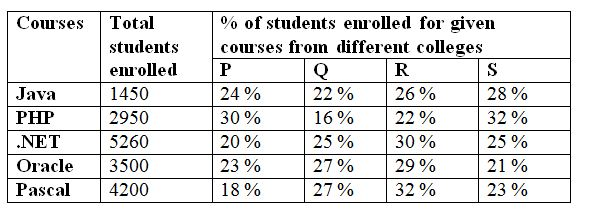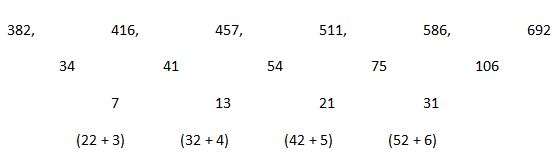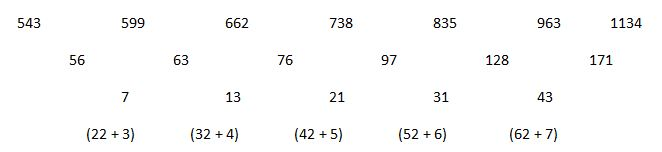# SBI PO Prelims Quantitative Aptitude Questions 2019 – Day 17

SBI PO 2019 Notification will be expected soon. It is one of the most expected recruitment among the banking aspirants. Every year the exam pattern for SBI PO has been changing. Depends upon the changing of exam pattern the questions are quite harder compare to the previous year. So the questions are in high level than the candidate’s assumption.

As per the latest trend, our IBPS Guide is providing the updated New Exam Pattern Quantitative Aptitude questions for SBI PO 2019 Day 17. Our Skilled experts were mounting the questions based on the aspirant’s needs. So candidates shall start your preparation and practice on daily basis with our SBI PO pattern quantitative aptitude questions 2019 day 17. Start your effective preparation from the right beginning to get success in upcoming SBI PO 2019.

[WpProQuiz 5296]

### Click Here for SBI PO Pre 2019 High-Quality Mocks Exactly on SBI Standard

Directions (1 – 5): The questions below are based on the given Series-I. The series-I satisfy a certain pattern, follow the same pattern in Series-II and answer the questions given below.

1)

I) 221, 238, 204, 255, 187, 272

II) 116 ………. 82. If 82 is nth term, then what value should come in place of (n + 3)rd term?

a) 184

b) 192

c) 166

d) 178

e) 150

2)

I) 458, 230, 61.5, 17.25, 12.15625

II) 398 …… 12. If 12 is nth term, then find the value of n?

a) 6th term

b) 4th term

c) 5th term

d) 7th term

e) 8th term

3)

I) 382, 416, 457, 511, 586, 692

II) 543, 599…….. 963. If 963 is nth term, then what value should come in place of (n + 1)th term?

a) 1066

b) 1088

c) 1192

d) 1134

e) 1050

4)

I) 12, 7, 9, 21, 88, 709

II) 44…….. 1733. If 1733 is nth term, then find the value of n?

a) 5th term

b) 6th term

c) 7th term

d) 8th term

e) 4th term

5)

I) 1, 4, 36, 576, 14400

II) 3.5 …….. 2016. If 2016 is nth term, then what value should come in place of (n + 1)th term?

a) 50400

b) 42600

c) 38800

d) 45200

e) 48600

Directions (Q. 6 – 10) Study the following information carefully and answer the given questions:

The following table shows the total number of students enrolled for different computer courses and the percentage of total number of students enrolled for given courses from different colleges.

Courses  Total students enrolled % of students enrolled for given courses from different colleges6) Total number of students enrolled for the courses of .NET and Pascal from the college P and S together is approximately what percentage of total number of students enrolled for the courses of Java and Oracle from the college Q and R together?

a) 155 %

b) 170 %

c) 140 %

d) 125 %

e) 115 %

7) Find the difference between the total number of students enrolled for the course .PHP and Pascal from the college P and Q together to that of total number of students enrolled for the course Oracle and .NET from the college R and S together?

a) 2178

b) 3292

c) 1548

d) 1396

e) None of these

8) Find the ratio between the total number of students enrolled for all the given courses from college R to that of college S?

a) 2360 : 2155

b) 3952 : 3787

c) 4963 : 4366

d) 3145 : 2782

e) None of these

9) Find the total number of students enrolled for all the given courses from college P?

a) 4172

b) 3846

c) 2945

d) 3257

e) None of these

10) Total number of students enrolled for the course Java and Pascal is approximately what percentage less than the total number of students enrolled for the course PHP and Oracle?

a) 12 % more

b) 12 % less

c) 35 % more

d) 35 % less

e) 4 % less

Directions (Q. 1 – 5):

Series I pattern:

221 is the first term

221 + 17 = 238

238 – 34 = 204

204 + 51 = 255

255 – 68 = 187

187 + 85 = 272

272 is 6th term

Series II pattern:

116 is the first term

116 + 17 = 133

133 – 34 = 99

99 + 51 = 150

150 – 68 = 82 (nth term)

82 + 85 = 167 (n + 1)th term

167 – 102 = 65 (n + 2)nd term

65 + 119 = 184 (n + 3)rd term

Series I pattern:

458 is the first term

(458/2) + 1 = 230

(230/4) + 4 = 61.5

(61.5/6) + 7 = 17.25

(17.25/8) + 10 = 12.15625

12.15625 is 5th term

Series II pattern:

398 is the first term

(398/2) + 1 = 200

(200/4) + 4 = 54

(54/6) + 7 = 16

(16/8) + 10 = 12

12 is 5th term

Series I pattern:

382 is the first term. Then 692 is 6th term.The difference of difference is, (22 + 3), (32 + 4), (42 + 5), (52 + 6),….

(Or)

The difference of difference of difference is, 6, 8, 10, 12,…

Series II pattern:

543 is first term.nth term is 963. Then (n + 1)th term is 1134.

Series I pattern:

12 is the first term

12*0.5 + 1 = 7

7*1 + 2 = 9

9*2 + 3 = 21

21*4 + 4 = 88

88*8 + 5 = 709

709 is 6th term

Series II pattern:

44 is the first term

44*0.5 + 1 = 23

23*1 + 2 = 25

25*2 + 3 = 53

53*4 + 4 = 216

216*8 + 5 = 1733

1733 is 6th term

Series I pattern:

1 is the first term

1*22 = 4

4*32 = 36

36*42 = 576

576*52 = 14400

14400 is 5th term

Series II pattern:

3.5 is the first term

3.5*22 = 14

14*32 = 126

126*42 = 2016 (nth term)

2016*52 = 50400 (n + 1)th term

Directions (Q. 6 – 10):

Total number of students enrolled for the courses of .NET and Pascal from the college P and S together

= > 5260*(45/100) + 4200*(41/100)

= > 2367 + 1722 = 4089

Total number of students enrolled for the courses of Java and Oracle from the college Q and R together

= > 1450*(48/100) + 3500*(56/100)

= > 696 + 1960 = 2656

Required % = (4089/2656)*100 = 153.95 % = 155 %

Total number of students enrolled for the course PHP and Pascal from the college P and Q together

= > 2950*(46/100) + 4200*(45/100)

= > 1357 + 1890 = 3247

Total number of students enrolled for the course Oracle and .NET from the college R and S together

= > 3500*(50/100) + 5260*(55/100)

= > 1750 + 2893 = 4643

Required difference = 4643 – 3247 = 1396

The total number of students enrolled for all the given courses from College R

= > 1450*(26/100) + 2950*(22/100) + 5260*(30/100) + 3500*(29/100) + 4200*(32/100)

= > 377 + 649 + 1578 + 1015 + 1344

= > 4963

The total number of students enrolled for all the given courses from College S

= > 1450*(28/100) + 2950*(32/100) + 5260*(25/100) + 3500*(21/100) + 4200*(23/100)

= > 406 + 944 + 1315 + 735 + 966

= > 4366

Required ratio = 4963 : 4366

The total number of students enrolled for all the given courses from the

College P

= > 1450*(24/100) + 2950*(30/100) + 5260*(20/100) + 3500*(23/100) + 4200*(18/100)

= > 348 + 885 + 1052 + 805 + 756

= > 3846

Total number of students enrolled for the course Java and Pascal

= > 1450 + 4200 = 5650

Total number of students enrolled for the course PHP and Oracle

= > 2950 + 3500 = 6450

Required % = [(6450 – 5650)/6450]*100 = 12.40 % = 12 % less